### Gravitational Physics

The legend of Issac Newton usually begins in an idyllic English countryside and features a falling apple. In this setting, he developed the trademark mixture of distilled reason and judicious intuition that has dominated theoretical physics in the subsequent centuries.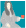Newton's most significant accomplishment was inventing a single model, which we now call Newtonian gravity, that gives rise to the dynamics of both:

• falling objects near Earth's surface;
• and planets in orbit.

These two types of motion were thought to be entirely distinct until Newton unified them within the framework of his theory.

Here, we will explore the insights that motivated Newton to propose his theory of gravity. We first trace out Newton's argument for the mass-dependence of his theory. Later, we will constrain its mathematical form with orbital data from Jupiter's moons.

# Explore: Mass Dependence

Newton studies an apple in his hand. He estimates that the mass of the apple is about 10% of the mass of his head.

How do the following gravitational forces compare?

• $F_{E\to H} =$ the force the Earth applies on Newton's head
• $F_{E\to A} =$ the force the Earth applies on the apple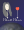Note: Newton is holding the apple on Earth's surface where the acceleration due to gravity is $g\approx\SI[per-mode=symbol]{10}{\meter\per\second\squared}.$

# Explore: Mass Dependence

Newton understood that forces come in pairs, which he expressed in his third law.

How do the following forces compare?

• $F_{H\to E}=$ the force Newton's head applies on the Earth
• $F_{A\to E}=$ the force the apple applies on the Earth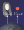# Explore: Mass Dependence

Here's the idea that set Newton apart from his contemporaries:

Newton speculated that any pair of massive objects applies a gravitational force on each other. In this sense, he characterized gravity as a universal force.

How do the following forces compare?

• $F_{H\to A}=$ the force Newton's head exerts on the apple
• $F_{A\to H}=$ the force the apple exerts on Newton's head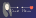# Explore: Mass Dependence

The last series of questions show us that the form of Newton's universal force law $F(m_1, m_2)$ needs to be

• proportional to each mass;
• symmetric with respect to each mass.

Which of these choices is a possible mass dependence of the law $F(m_1, m_2)$?

# Explore: Mass Dependence

This brand of model building through intuitive reasoning can only take us so far; at some point, any physical theory needs to appeal to observations and experiments.

Newton suspected that the force of gravity between two objects would depend not only on their masses, but also on their separation $r.$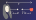Of course, Newton didn't have the means to leave Earth's surface to test how gravity's strength varies away from the surface, so he turned his head skyward, using observations of celestial bodies to justify his theory.

In the following quiz, we are going to make a string of deductions in the vein of Newton's own reasoning to find the complete form of his gravitational law.

×